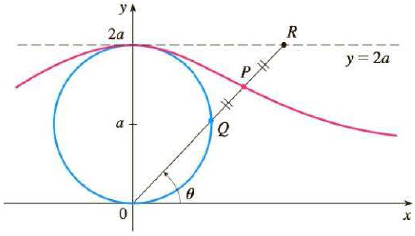Chapter 10, Problem 57RE

Chapter
Section
Textbook Problem

# In the figure the circle of radius a is stationary, and for every θ, the point P is the midpoint of the segment QR. The curve traced out by P for 0 < θ < π is called the long-bow curve. Find parametric equations for this curve.To determine

To find: The parametric equation of the given curve.

Explanation

Given:

The polar equation of the circle is as follows.

r=2asinθ (1)

The polar equation for the variable x is as follows.

x=rcosθ (2)

The polar equation for the variable y is as follows.

y=rsinθ (3)

Calculation:

Substitute equation (1) in (2) we get,

x=2asinθcosθ (4)

Substitute equation (1) in (3) we get,

y=2asin2θ (5)

The coordinate of point Q is (x1,y1)=(2asinθcosθ,2asin2θ) .

Determine the coordinates of point R.

From the diagram given in the given in the question,

y=2a (6)

Substitute equation (3) in (6) to get the below equation.

2a=rsinθ

r=2asinθ (7)

Substitute equation (7) in (2).

x=2asinθcosθ

x=2acotθ (8)

The coordinate of point R is (x2,y2)=(2acotθ,2a)

### Still sussing out bartleby?

Check out a sample textbook solution.

See a sample solution

#### The Solution to Your Study Problems

Bartleby provides explanations to thousands of textbook problems written by our experts, many with advanced degrees!

Get Started

#### 12. Find .

Mathematical Applications for the Management, Life, and Social Sciences

#### True or False: converges.

Study Guide for Stewart's Multivariable Calculus, 8th

#### True or False: x2 + 6x + 2y = 1 defines y as a function of x.

Study Guide for Stewart's Single Variable Calculus: Early Transcendentals, 8th